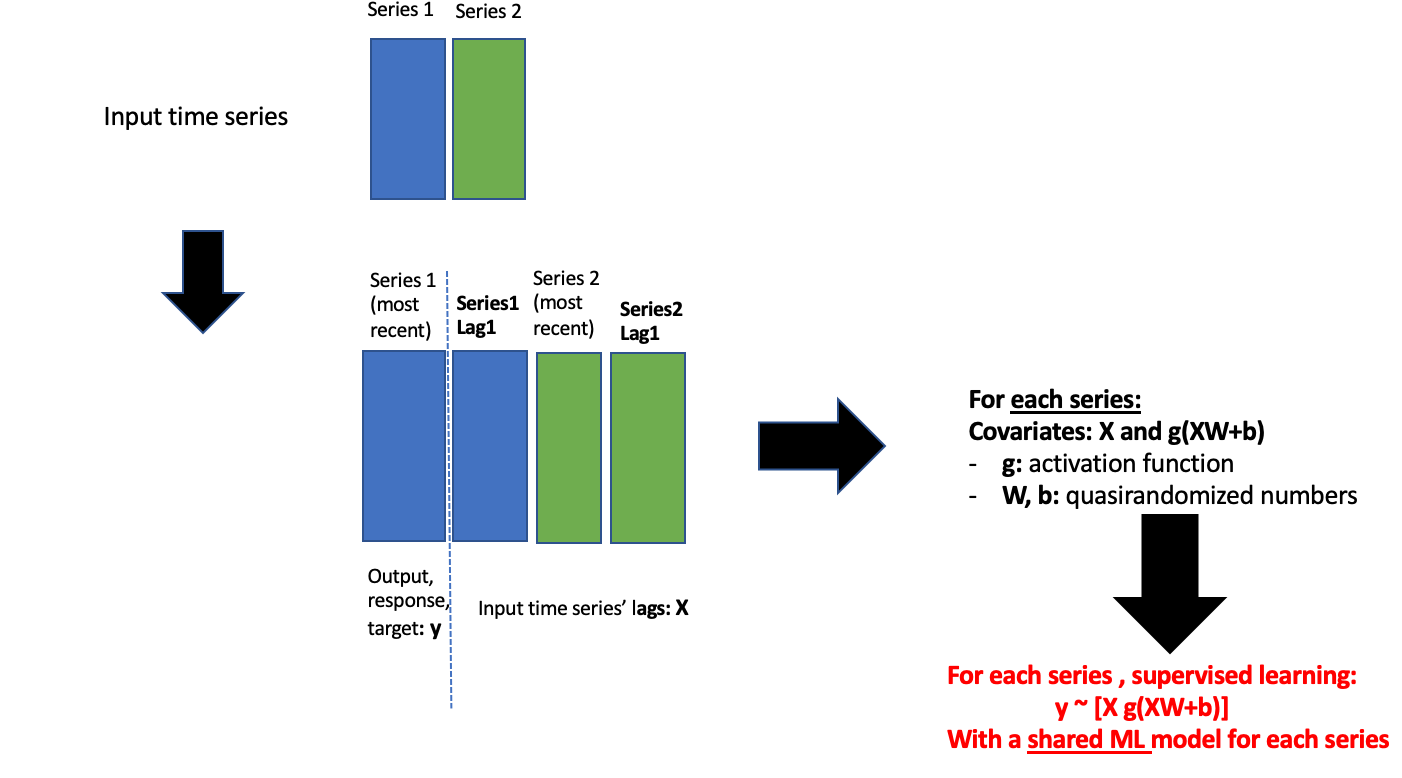Update 2023-09-27: conda users, v0.13.0 of nnetsauce is now available for Linux and macOS. For Windows, please use WSL2.

As I said a few years ago, nnetsauce.MTS is a family of univariate/multivariate time series forecasting models that I was supposed to present at R/Finance 2020 (this post is 100% Python) in Chicago, IL. But the COVID-19 decided differently.

The more I thought about it, namely nnetsauce.MTS (still doesn’t have a more glamorous name), the more I thought ‘It’s kind of weird…‘. Why? Because in the statistical learning procedure, all the input time series models share the same hyperparameters. Today, I think nnetsauce.MTS it’s not quite different from a multi-output regression (regression models for predicting multiple responses, based on covariates), and it seems to be working well empirically, as shown below. No grandiose state-of-the-art (SOTA for the snobs) claims here, but I think that with the high number of possible model inputs (actually, any regression Estimator having fit and predict methods), you could cover a lot of space.

You can read this post if you want to understand how it works (but avoid the ugly graph at the end, the ones presented here are hopefully more compelling). Pull requests and (constructive) discussions are welcome as usual.

In the examples presented here, I focus on uncertainty quantification:

• simulation-based, using Kernel Density Estimation of the residuals
• a Bayesian approach, even though ‘Bayesianism’ is in hot water these days. Its subjectivity? I must admit that choosing a prior distribution is quite an interesting (interpret ‘interesting’ here as you want, I mean both good and bad) experiment. But ‘Bayesianism’, Gaussian Processes in particular, works quite well in settings such as hyperparameters tuning (I hope the code still works) for example

Conformal prediction, the new cool kid on the uncertainty quantification block, will certainly be included in future versions of the tool.

Contents

• 0 - Install and import packages + get data
• 1 - Simulation-based forecasting using Kernel Density Estimation
• 1 - 1 With Ridge regression
• 1 - 2 With Random Forest
• 2 - Bayesian Forecasting
• Appendix

You can also download this notebook from GitHub, which follows the same plan.

# 0 - Install and import packages + get data

Installing nnetsauce (v0.13.0) with pip:

pip install nnetsauce


Installing nnetsauce (v0.13.0) using conda:

conda install -c conda-forge nnetsauce


Installing from GitHub:

pip install git+https://github.com/Techtonique/nnetsauce.git


Import the packages in Python:

import nnetsauce as ns
import numpy as np
import pandas as pd
from sklearn.linear_model import Ridge, BayesianRidge
from sklearn.ensemble import RandomForestRegressor
from time import time


Get data:

url = "https://raw.githubusercontent.com/thierrymoudiki/mts-data/master/heater-ice-cream/ice_cream_vs_heater.csv"

# ice cream vs heater (I don't own the copyright)
df.set_index('Month', inplace=True)
df.index.rename('date')

df = df.pct_change().dropna()

idx_train = int(df.shape*0.8)
idx_end = df.shape
df_train = df.iloc[0:idx_train,]


# 1 - Simulation-based forecasting using Kernel Density Estimation

## 1 - 1 With Ridge regression

regr3 = Ridge()
obj_MTS3 = ns.MTS(regr3, lags = 3, n_hidden_features=7, #IRL, must be tuned
replications=50, kernel='gaussian',
seed=24, verbose = 1)
start = time()
obj_MTS3.fit(df_train)
print(f"Elapsed {time()-start} s")

res = obj_MTS3.predict(h=15)
print("\n")
print(f" Predictive simulations #10: \n{obj_MTS3.sims_}")
print("\n")
print(f" Predictive simulations #25: \n{obj_MTS3.sims_}")

obj_MTS3.plot("heater")
obj_MTS3.plot("ice cream")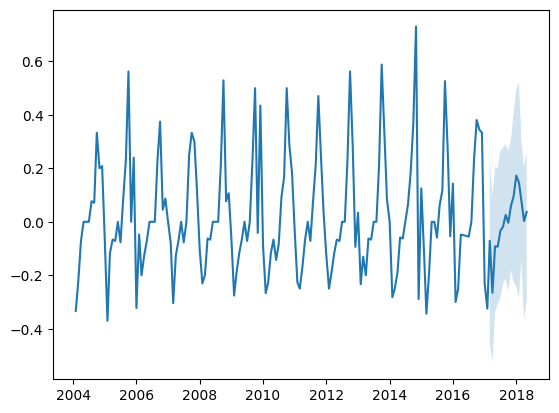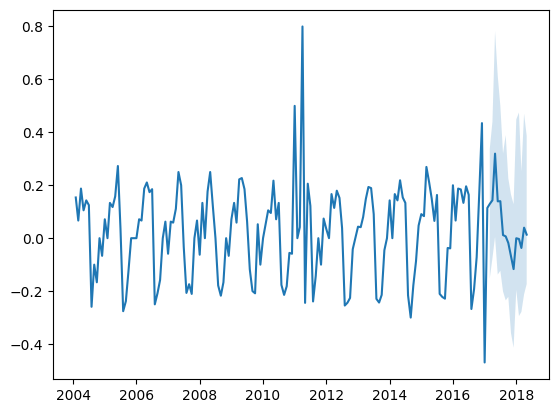## 1 - 2 With Random Forest

regr3 = RandomForestRegressor(n_estimators=250)
obj_MTS3 = ns.MTS(regr3, lags = 3, n_hidden_features=7, #IRL, must be tuned
replications=50, kernel='gaussian',
seed=24, verbose = 1)
start = time()
obj_MTS3.fit(df_train)
print(f"Elapsed {time()-start} s")

res = obj_MTS3.predict(h=15)
print("\n")
print(f" Predictive simulations #10: \n{obj_MTS3.sims_}")
print("\n")
print(f" Predictive simulations #25: \n{obj_MTS3.sims_}")

obj_MTS3.plot("heater")
obj_MTS3.plot("ice cream")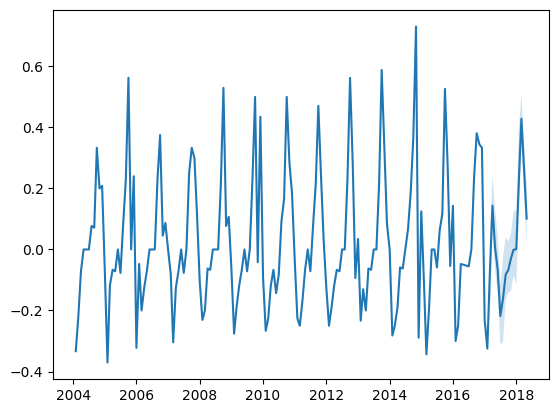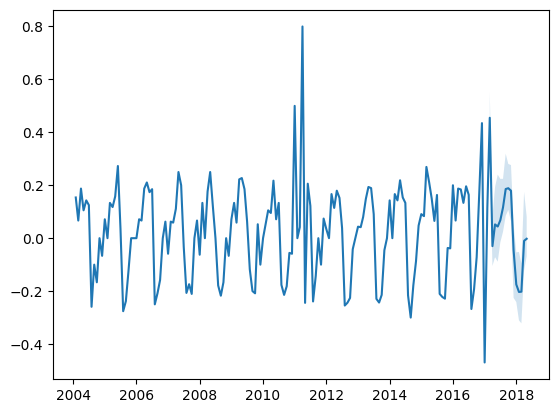# 2 - Bayesian Forecasting

regr4 = BayesianRidge()
obj_MTS4 = ns.MTS(regr4, lags = 3, n_hidden_features=7, #IRL, must be tuned
seed=24)
start = time()
obj_MTS4.fit(df_train)
print(f"\n\n Elapsed {time()-start} s")

res = obj_MTS4.predict(h=15, return_std=True)

obj_MTS4.plot("heater")
obj_MTS4.plot("ice cream")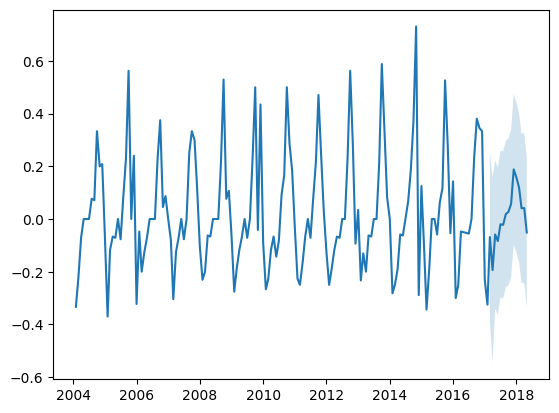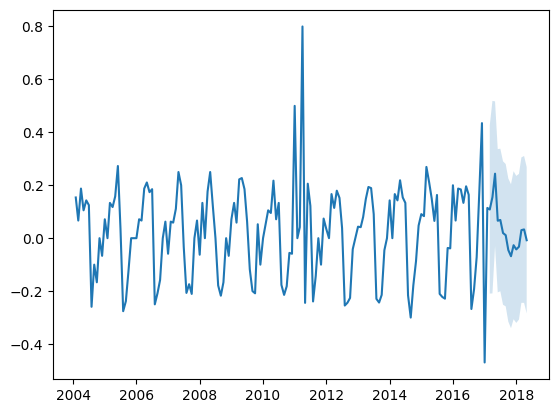# Appendix

How does this family of time series forecasting models works?